To use a formula in Excel, you must follow the process described below. First, select any cell and type in it the equal symbol (=) to tell the program that we are going to work with a formula: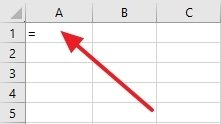Starting a formula

Then, complete the formula. To do so, there are different methods available. You can write a series of numbers and operators: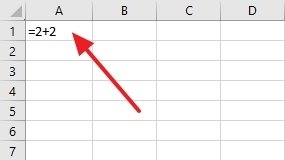Simple operation in a cell

Use the mouse to select other cells, inserting an operator between them, and clicking on a third cell (this one is where the result will be displayed):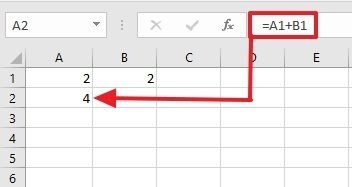Adding up a range in a third cel

Typing a letter to choose from a list of spreadsheet functions: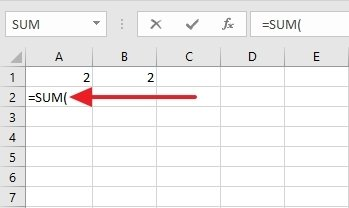Formula based on a function

If you choose this method, you will have to complete the formula using numbers, cell identifiers, and operators by pressing Enter. If you want to complete a formula that uses a function, enter the required information (usually a description of what it does), and press Enter: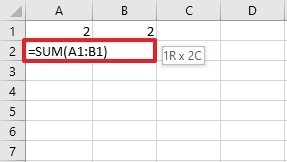Function completed with a range of cells

Formulas are equations that let the user perform automatic calculations, return information, manipulate the contents of other cells, and so on. This is a good way to automate some of the work since it lets us cross-reference data and obtain the result offered by the combination of the information.

A formula is composed of the following elements:

• Constants or text: constants are a number, while text must be enclosed in quotation marks.
• Cell references: they can replace constants and are references to the cells that have the value we want to use.
• Operators: they are the symbols of the mathematical operators (addition, subtraction, multiplication, and so on).
• Excel functions: formulas that let us use predefined functions used by this software.